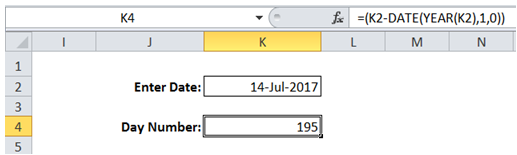## Day number of the year in Excel

To do it in Excel, here is the answer:

a) Enter the formula =(K2-DATE(YEAR(K2),1,0)) where K2 corresponds to "Date" input for which day number is required.

To calculate the day number the first ate of the year has to be subtracted from specified date.

Date corresponding to the first day of the year has the same year as the specified date with month being 1 and day being 0 (representing start of year).

The function (DATE(YEAR(K2),1,0) returns the first day corresponding to the year of the Date specified. This is subtracted from the date specified to get "Day number".

The "Day number" field (cell K4) has to be formatted as number.